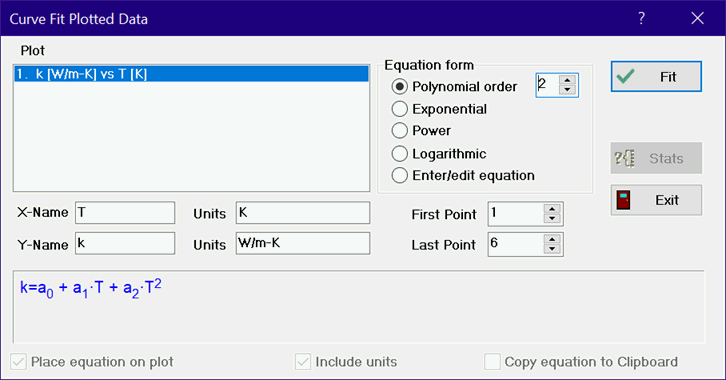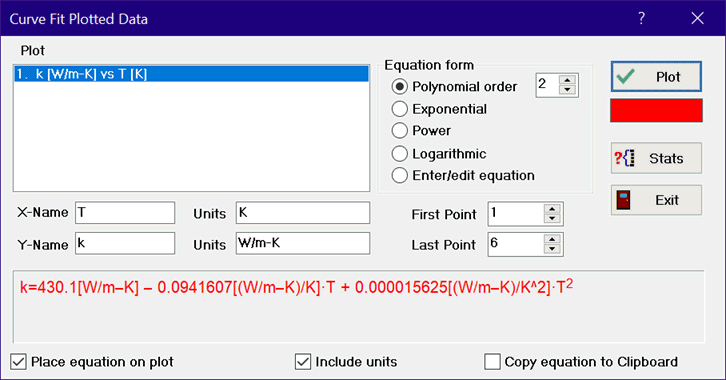Contents - Index

Curve Fit

The Curve Fit command will find the best fit of a smooth curve through a previously plotted set of data points using unweighted least squares.  Chose the data to be fitted from the list of plots at the left.  Note that data can be plotted from the ParametricLookupArrays, or Integral table with the New Plot or Plot_Overlay_Plot commands.  The first and last point will be displayed for the selected plot.  You can change these values if you wish to plot a subset of the data.Select the form of the curve fit by clicking the appropriate radio button.  A sample of the equation form will appear in blue in the box at the bottom of the dialog window.  The first four buttons correspond to commonly used equation forms for which linear least squares can be used to determine the unknown coefficients.  The Enter/Edit Equation button allows you to enter any equation form or to edit a previously entered equation.  The equation you enter may be linear or nonlinear in the unknown parameters.  You will have to supply guess values and (optionally) bounds for the unknown parameters.

Click the Fit button (or press the Enter key).  The fitted equation will be displayed in the box at the bottom of the dialog window.A Stats button will appear.  Click the Stats button if you wish to see statistical information relating to the curve fit, such as the standard error of the parameters and the root mean square error of the fit.  The Fit button will now have changed into the Plot button.  Click the Plot button if you wish to have the curve fit equation overlaid on your plot.  If the Plot Legend check box is selected, a legend containing the equation will be created and displayed on the plot.  The curve fit equation will be copied to the Clipboard if the To Clipboard check box is selected when either the Plot or Exit button is selected.

The curve fit command will regress any plotted variable as a linear or non-linear function of any other variable.  The Linear Regression command in the Tables menu provides linear regression capability for one to nine independent variables.